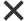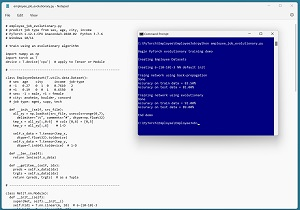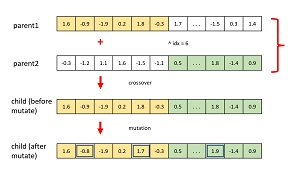News

### Researchers Explore Evolutionary Algorithms for Neural Network Training

Compared to standard neural network training techniques that are based on mathematical backpropagation, evolutionary training allows more complex neural architectures.Machine learning researchers at Microsoft have demonstrated a technique for training deep neural networks that is based on biological mechanisms of genetic crossover and mutation. The technique is known as an evolutionary algorithm. Compared to standard neural network training techniques that are based on mathematical backpropagation, evolutionary training allows more complex neural architectures.

The Problem
A standard deep neural network can be thought of as a very complex mathematical function. Suppose you have data that looks like:

```-1  0.29  1 0 0  0.67500  2
1  0.33  0 1 0  0.58000  1
-1  0.25  0 1 0  0.63500  0
1  0.42  0 0 1  0.71000  2
. . .```

The data represents employees. The fields are sex (male = -1, female = +1), age (divided by 100), city (Anaheim = 100, Boulder = 010, Concord = 001), income (divided by 100,000), and job type (0 = management, 1 = sales, 2 = technical). The goal is to predict job type from the other four variables.

One possible neural architecture for this problem is 6-(10-10)-3. This means there are six input nodes, two hidden layers each with 10 nodes, and three output nodes. After the network has been trained, an input of (-1, 29, 1,0,0, 0.65000) might produce an output that looks like (0.27, 0.62, 0.11). The output values are called pseudo-probabilities and they represent the likelihood of each of the three possible job types. Therefore, this output would be interpreted as a prediction of 1 = sales because the value at location , 0.62, is the largest.[Click on image for larger view.] Figure 1: Training Using Backpropagation and Evolution

The screenshot in Figure 1 shows an example of a network being trained using both standard backpropagation and using an evolutionary algorithm. In this example, the results are comparable -- both models give roughly 85 percent prediction accuracy.

The number of input nodes and output nodes are determined by the problem to be solved. The number of hidden layers and the number of nodes in each hidden layer are called hyperparameters which means they're values that must be determined through experimentation guided by experience and intuition. More hidden layers and more nodes in each layer creates a more complex neural network.

For a 6-(10-10)-3 neural network, there are (6 * 10) + 10 + (10 * 10) + 10 + (10 * 3) + 3 = 213 constants called weights and biases. Each weight and bias is just a number, typically between about -5.0 and +5.0. The values of the weights and biases, along with a set of input values, determine the output of a neural network. The process of finding good values for the weights and biases is called training the network.

Training a neural network is accomplished by using a set of training data that has known input values and known, correct output values. A training algorithm searches through different combinations of values of the weights and biases, looking for a set of values where predicted outputs closely matched the known correct outputs in the training data.

Evolutionary Algorithms
Training modern neural networks is usually accomplished using a technique that is based on mathematical calculus called backpropagation (originally spelled back-propagation). There are many variations of backpropagation with names such as stochastic gradient descent (SGD) and Adam (adaptive moment estimation). Backpropagation was invented in the 1960s but didn't become the standard technique for training neural networks until about 2012.

Backpropagation training techniques are efficient and effective, but backpropagation has limitations. Specifically, for a network to be trained using backpropagation, all parts of the network must be mathematically differentiable. Training a neural network using an evolutionary algorithm works for any kind of neural network architecture.

Expressed in pseudo-code, evolutionary training is:

```
create a population of n solutions
loop many generations
select two parents from population
create a child solution from parents
mutate child slightly
replace a weak solution in pop with child
end-loop
return best solution found

```

The diagram in Figure 2 illustrates the key ideas. Each parent is a possible solution to network training -- each parent has 213 values which are weights and biases for the network being trained. A crossover point is selected and a child is created from the left half of one parent and the right half of the other parent. The child is mutated slightly by adjusting randomly selected values. The new child is placed into the population of solutions, replacing a weak solution. After many generations, a good solution will evolve.[Click on image for larger view.] Figure 2: Evolutionary Crossover and Mutation

What Does It Mean?
The Pure AI editors spoke with Dr. James McCaffrey from Microsoft Research. McCaffrey commented, "The idea of evolutionary algorithms for training neural networks has been looked at for many years. In general, for small and simple neural networks, the advantage gained by evolutionary training wasn't worth the added effort."

McCaffrey added, "But the new generation of large neural networks, especially those for natural language processing and image processing, when combined with greatly increased processing power, motivated us to take a new look at evolutionary algorithms for training."

McCaffrey further commented, "An area which hasn't been thoroughly explored is creating neural architectures that have non-differentiable components. Such architectures can be trained using evolutionary algorithms and might enable us to look at entirely new types of problems."